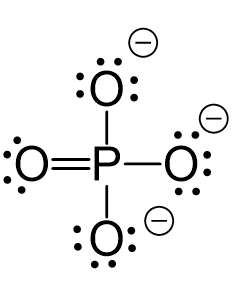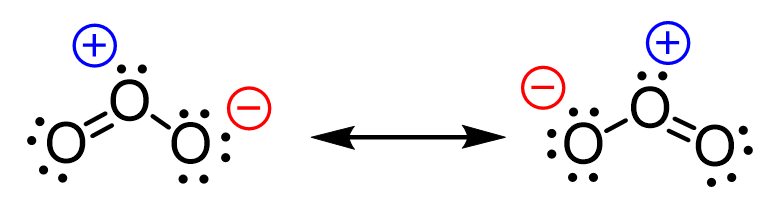## General Chemistry

In the previous post, we talked about the covalent bond and mentioned that sometimes, depending on the number of bonds, the given atom might have either a positive or a negative charge.

For example, the oxygen in water has no charge, however, in the hydroxide ion, which is the most common species acting as a base, bears a negative charge:Let’s understand where this charge is coming from. Any charge on an atom is a result of an imbalance between the number of the protons (positive charges) and the number of electrons (negative charges). Now, the number of protons does not change, otherwise, we’ll have a different element. So, it must be the number of electrons that causes the charge imbalance. We know that it is only the valance electrons that participate in bonding and chemical reactions because the inner electrons are too tightly bound to the nucleus.

# Calculating the Formal Charge

To determine whether the atom in a molecule or an ion is going to be charged, we need to compare the number of valence electrons in an isolated atom with the number of electrons that are associated with the same atom in a Lewis structure.

When the atom is by itself, the number of electrons associated with the is simply its number of valence electrons. (Again, we do not count the inner electrons.) When the atom is in a molecule, it is surrounded by two types of electrons: the bonding electrons and nonbonding electrons (if any). The nonbonding electrons belong to the atom as it does not share them with other atoms. The bonding electrons, however, are shared with other atom(s), and therefore, we need to divide them by two to count them toward the atom.

These are the assigned electrons of the atom – the electrons that it owns. So, it owns all the nonbonding electrons plus half of the bonding electrons. Now, the difference between the valence electrons and the sum of the electrons assigned to the atom is what defines whether the atom has a formal charge or not.In the case of water, the oxygen owns 6 electrons, 4 of which are the lone pairs and 2 that are half of the 4 bonding electrons. Together they make 6 electrons which is equal to the number of the valence electrons and therefore, the oxygen has no formal charge. On the other hand, in the hydroxide ion, it has 7 electrons – 6 from the 3 lone pairs, and 1 that half the 2 bonding electrons. The difference is -1 and that is the formal charge of the oxygen.

# The Formal Charge of Ions

Let’s consider the phosphate ion, PO43-. It has a formal charge of -3 which is the sum of -1 charge coming from each oxygen. And we can see from the Lewis structure of the ion why the oxygen is negatively charged. The reason is that they only have one bond and instead of two lone pairs like in water, they have three lone pairs:# Positive Formal Charge

Atoms can also be positively charged if they do not have enough electrons to match the number of valence electrons. For example, below is an acceptable Lewis structure of ozone (O3):

The oxygen in the middle is positively charged because although it has an octet, it still owns less than 6 electrons:Notice that unlike the oxygen on the right, the one on the left has no formal charge since it follows the standard valency of oxygen. That is 2 bonds and 2 lone pairs.

So, the question is, can the electrons be redistributed such that the negative charge is passed on to this oxygen?And the answer is yes, they can and that is what we call resonance structures. These are different Lewis structures of the same compound where only the distribution of the electrons is changed while the connectivity of the atoms remains the same. We will discuss the resonance structures as well as they are used in organic chemistry in the next article.

Check Also

Check this 90-question, Multiple-Choice Quiz on Chemical Bonding: